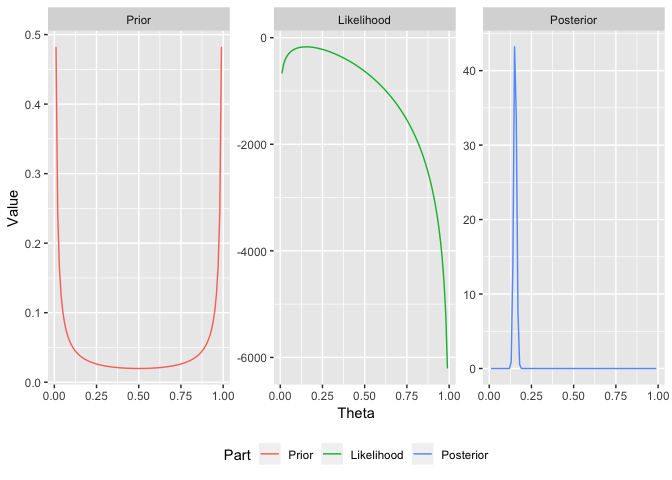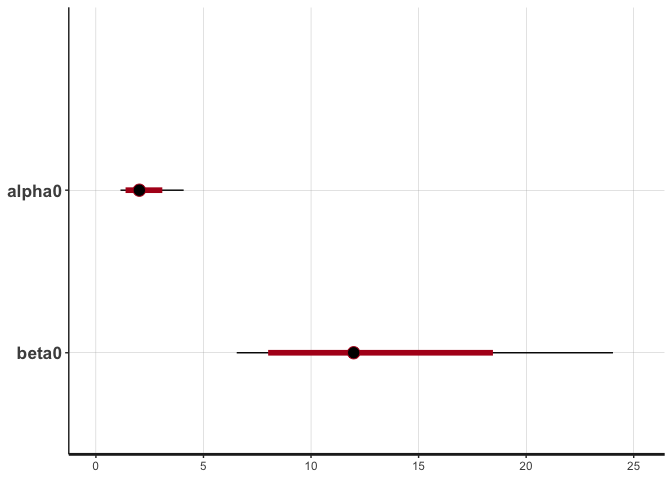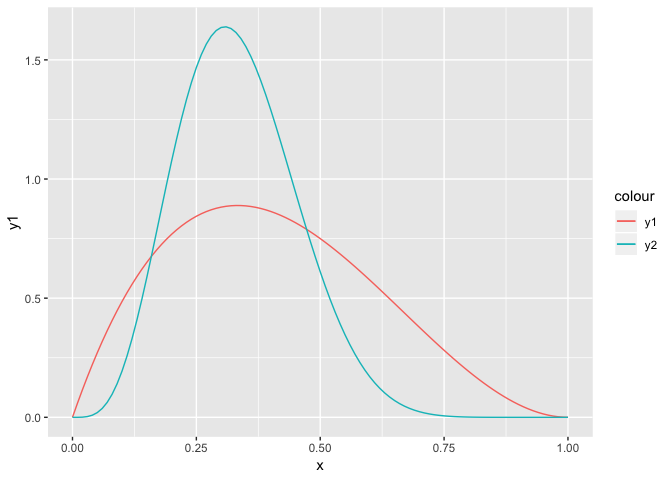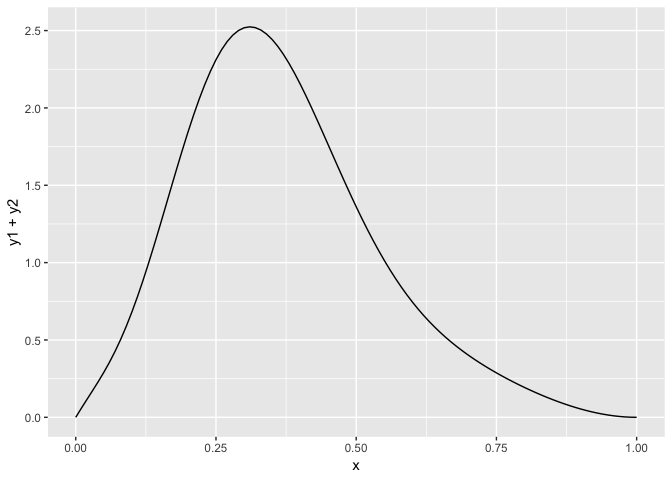require(dplyr)
require(knitr)
require(ggplot2)
require(dirichletprocess)
require(rstan)
require(tidyr)


One of the main benefits of my R package dirichletprocess is the ability to drop in the objects it creates as components of models. In this blog post I will show you how you can use a Dirichlet process as a prior distribution of a parameter in a Bayesian model.

### Rats!

The data we will be using consists of 71 experiments where $$N$$ rats are given a drug and $$y$$ are found to have developed tumours.

rats %>% sample_n(4) %>% kable

y N
2 24
6 22
3 27
1 18

We want to model $$\theta = \frac{y}{N}$$, which is the rate of tumours in each experiment.

### The Basic Bayesian Model

In the most basic model, we can assume that the the rats with tumours are Binomially distributed at a rate of $$\theta$$, which can be written as:

\begin{aligned} y_i & \sim \text{Binomial} (N_i, \theta _i), \\ \theta_i & \sim \text{Beta} (\alpha _0, \beta _0). \\ \end{aligned}

We then assume that there is only one $$\theta$$ and it applies to each experiment. As this is a conjugate model, we can easily apply Bayes theorem and arrive at a analytical posterior.

\begin{aligned} \theta & \mid y_i, N_i \sim \text{Beta} (\alpha , \beta), \\ \alpha & = \alpha_0 + \sum _{i=1} ^n y_i, \\ \beta & = \beta_0 + \sum _{i=1} ^n N_i - \sum _{i=1} ^n y_i. \end{aligned}

Which we translated into R as:

alpha0 <- 0.01
beta0 <- 0.01

alphaPosterior <- alpha0 + sum(rats$y) betaPosterior <- beta0 + sum(rats$N) - sum(rats$y) thetaDraws <- rbeta(1000, alphaPosterior, betaPosterior)  We’ve chosen uninformative prior values for $$\alpha_0$$ and $$\beta_0$$. qplot(thetaDraws, geom="histogram", binwidth=0.01)So we estimate the rate of tumours to be about 15%. Now for the classic Bayesian graphic which shows the prior, the likelihood and the resulting posterior. xGrid <- seq(0.01, 1-0.01, 0.01) bayesFrame <- data.frame(Theta=xGrid, Prior = dbeta(xGrid, alpha0, beta0), Likelihood = vapply(xGrid, function(x) sum(dbinom(rats$y, rats$N, x, log=T)), numeric(1)), Posterior = vapply(xGrid, function(x) dbeta(x, alphaPosterior, betaPosterior), numeric(1))) bayesFrame %>% gather(Part, Value, -Theta) -> bayesFrameTidy bayesFrameTidy$Part <- factor(bayesFrameTidyPart, levels=c("Prior", "Likelihood", "Posterior")) ggplot(bayesFrameTidy, aes(x=Theta, y=Value, colour=Part)) + geom_line() + facet_wrap(~Part, scales="free") + theme(legend.position = "bottom")This model though is too simple, we pool all the results and assume that there is one $$\theta$$ that applies to all the experiments. A better model would be to allow for variation across the experiments. Also, we thought the prior was uninformative, its actually incredibly informative at the boundaries. Can we find better values of $$\alpha _0$$ and $$\beta _0$$. ### A Hierarchical Model In this model, we are now assuming that each experiment has its own $$\theta _i$$. Furthermore, we place a prior on $$\alpha _0$$ and $$\beta _0$$ we allow them to vary in the inference process aswell. \begin{aligned} \theta _i \mid \alpha _0 , \beta _0 & \sim \text{Beta} (\alpha _0, \beta _0), \\ y_i \mid N_i , \theta _i & \sim \text{Binomial} (N_i, \theta _i ). \end{aligned} So instead of setting $$\alpha _0$$ and $$\beta_0$$ to specific values, we allow them to vary and find the most suitable values. But we loose the conjugacy and have to use Stan to sample from the model. We build the model in Stan view the .stan here and sample form the posterior distribution. stanModel <- stan_model("rats_hyper.stan") stanData <- list() stanDatay <- rats$y stanData$N <- rats$N stanData$n <- nrow(rats)

smpls <- sampling(stanModel, stanData, chains=4, iter=5000, cores=2)

plot(smpls, par=c("alpha0", "beta0"))Here we can see that there is quite a bit of uncertainty around the $$\beta_0$$ value. We extract the samples to use later.

as.data.frame((rstan::extract(smpls, pars=c("mu", "nu")))) -> postParams
as.data.frame((rstan::extract(smpls, pars=c("alpha0", "beta0")))) -> postParamsAlt


### Dirichlet Process

What if we relaxed the assumption that the prior on $$\theta$$ needs to be parametric. Instead, what if we represented our prior on $$\theta$$ as an infinite mixture model, and updated it with each posterior draw.

\begin{aligned} \theta _i & \sim F \\ F & = \sum _{k=1} ^\infty w_k k(\theta \mid \phi _k) \end{aligned}

where $$k$$ is just the $$\text{Beta}$$ distribution and $$\phi _k$$ represents that distributions parameters.

To help visualise this lets consider two components of equal weight,

wk <- c(0.5, 0.5)
phik_alpha <- c(2, 5)
phik_beta <- c(3, 10)

xGrid <- seq(0, 1, by=0.01)

frameF <- data.frame(x=xGrid,
y1 = wk * dbeta(xGrid, phik_alpha, phik_beta),
y2 = wk * dbeta(xGrid, phik_alpha, phik_beta))

ggplot(frameF) +
geom_line(aes(x=x, y=y1, colour="y1")) +
geom_line(aes(x=x, y=y2, colour="y2"))Then when you combine them you get:

ggplot(frameF, aes(x=x, y=y1+y2)) + geom_line()Now if we let the number of components got to infinity we can make the distribution whatever shape we want.

We want to apply the same type of logic to learn an infinite component mixture model. This means that the prior will learn the best fitting distribution.

For each iteration, we guess the prior distribution using the PosteriorClusters function. This returns a list of weights and parameters. We then sample from the weights for each point, to guess what cluster it belonged too. Then using these cluster parameters we can draw a value of $$\theta$$. This is repeated for each point to produce a new value for $$\theta _i$$ for all $$i$$. We then pass these new values back into the dp object using ChangeObservations and Fit to update the cluster parameters and weights. The process is repeated for as many iterations as needed.

FIT <- FALSE
its <- 1500

if(FIT){

print("Starting Fit")

1,
mhStep = c(0.002, 0.005),
alphaPrior = c(2, 0.5))

dp$numberClusters <- nrow(rats) dp$clusterLabels <- seq_len(nrow(rats))
dp$clusterParameters <- PriorDraw(dp$mixingDistribution, nrow(rats))
dp$pointsPerCluster <- rep_len(1, nrow(rats)) dp <- Fit(dp, 1) postFuncEval <- matrix(ncol=its, nrow=length(xGrid)) muPostVals <- matrix(ncol=its, nrow=nrow(rats)) nuPostVals <- matrix(ncol=its, nrow=nrow(rats)) pb <- txtProgressBar(max=its, width=50, char="-", style=3) for(i in seq_len(its)){ postClusters <- PosteriorClusters(dp) postFuncEval[,i] <- PosteriorFunction(dp)(xGrid) wk <- sample.int(length(postClusters$weights),
nrow(rats),
replace = T,
prob = postClusters$weights) muPost <- postClusters$params[][,,wk]
nuPost <- postClusters$params[][,,wk] aPost <- muPost * nuPost bPost <- (1-muPost) * nuPost muPostVals[,i] <- muPost nuPostVals[,i] <- nuPost newTheta <- rbeta(nrow(rats), aPost + rats$y, bPost + rats$N - rats$y)

dp <- ChangeObservations(dp, newTheta)
dp <- Fit(dp, 100, updatePrior = T, progressBar = F)

setTxtProgressBar(pb, i)

}

saveList <- list()
saveList$muPostVals <- muPostVals saveList$nuPostVals <- nuPostVals
saveList$postFuncEval <- postFuncEval saveRDS(saveList, "dpPrior.RDS") } else { print("Fit from Cache") saveList <- readRDS("dpPrior.RDS") saveList$muPostVals -> muPostVals
saveList$nuPostVals -> nuPostVals saveList$postFuncEval -> postFuncEval
}


Once the fitting is finished we collect the resulting posterior samples of the prior parameters.

dirichletParamsMu <- data.frame(Value = c(muPostVals[,-(1:its/2)]))
dirichletParamsMu$Parameter= "Mu" dirichletParamsNu <- data.frame(Value = c(nuPostVals[,-(1:its/2)])) dirichletParamsNu$Parameter="Nu"

dirichletParams <- bind_rows(dirichletParamsMu, dirichletParamsNu)

ggplot(dirichletParams, aes(x=Value)) + geom_density() + facet_wrap(~Parameter, scales="free")ggplot(dirichletParams, aes(x=log(Value))) + geom_density() + facet_wrap(~Parameter, scales="free")Each peak in the density shows a cluster that is sampled in the Dirichlet process. From here we can see that there is only one real cluster for $$\mu$$, but for $$\nu$$ there are multiple cluster values that are sampled. It easier to see on the log scale.

nclusters <- apply(muPostVals[, -(1:its/2)], 2, function(x) length(unique(x)))
qplot(x=nclusters, geom="histogram", binwidth=1)Here we can see that whilst the vast majority of the time it finds 1 cluster, there is plenty of samples where there is more than one cluster.

### Resulting Priors

Now we compare the priors of the hierarchical model and that of the Dirichlet model.

apply(postParamsAlt, 1, function(x) dbeta(xGrid, x, x)) -> hierEval

hierEvalFrame <- data.frame(x=xGrid,
Mean=rowMeans(hierEval),
LQ = apply(hierEval, 1, quantile, probs=0.025),
UQ = apply(hierEval, 1, quantile, probs=1-0.025),
Model = "Hierarchical")

funcMean <- rowMeans(postFuncEval[,-(1:its/2)])

dirEvalFrame <- data.frame(x=xGrid,
Mean = rowMeans(postFuncEval),
LQ = apply(postFuncEval, 1, quantile, probs=0.025),
UQ = apply(postFuncEval, 1, quantile, probs= 1 - 0.025),
Model = "Dirichlet")

allEvalFrame <- bind_rows(hierEvalFrame, dirEvalFrame)

ggplot(allEvalFrame) +
geom_ribbon(aes(x=x, ymin=LQ, ymax=UQ, colour=Model, fill=Model), alpha=0.2) +
geom_line(aes(x=x, y=Mean, colour=Model)) +
facet_grid(~Model) +
scale_x_continuous(limits = c(0.01, 1-0.01)) +
guides(colour = FALSE, fill=FALSE)So the differences in the resulting priors are very negligible to the eye. Reassuringly, we can see that the Dirichlet model has resulted in the same shape as the hierarchical model, which in this case, suggests that our data can be modelled without the need for a non-parametric prior.

You could perform the standard model checking techniques to find out if the Dirichlet prior performs better. I’ll leave that as an exercise for the reader. This blog post was about showing how the dirichletprocess package can be easily used to drop non-parametric components of models into problems.

If you like what you see download dirichletprocess from CRAN or head over to the Github here for the latest dev version.